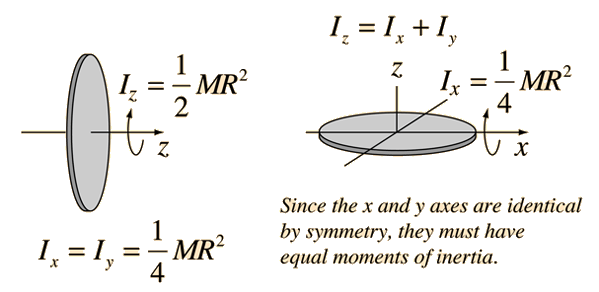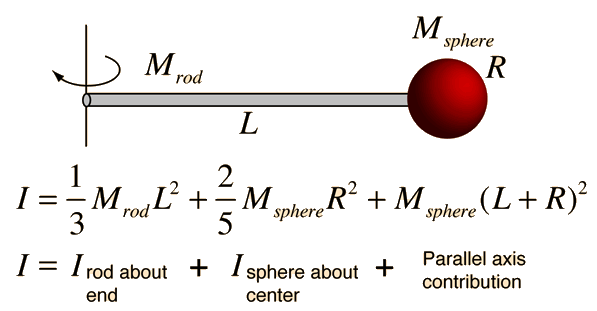# Moment of Inertia: Thin Disk

The moment of inertia of a thin circular disk is the same as that for a solid cylinder of any length, but it deserves special consideration because it is often used as an element for building up the moment of inertia expression for other geometries, such as the sphere or the cylinder about an end diameter. The moment of inertia about a diameter is the classic example of the perpendicular axis theorem For a planar object:Index

Moment of inertia concepts

 HyperPhysics***** Mechanics ***** Rotation R Nave
Go Back

# Superposition of Moments of Inertia

The moment of inertia of a composite object can be obtained by superpositon of the moments of its constituent parts. The Parallel axis theorem is an important part of this process. For example, a spherical ball on the end of a rod:For rod length L = m and rod mass = kg,
sphere radius r = m and sphere mass = kg:
 Irod about end = kg m2 Isphere about center = kg m2 Parallel axis contribution = kg m2
The composite moment of inertia is given by the sum of the contributions shown at left. The moment of inertia is
I = kg m2

It may be instructive to compare this moment of inertia with that of a rod or sphere alone. If the total mass of kg were concentrated in the sphere, the moment of inertia would be
I(sphere) = kg m2.

If the entire mass were concentrated in the rod and it was extended to the location of the center of the sphere at m, then the moment of inertia would be
I(rod) = kg m2.
Index

Moment of inertia concepts

 HyperPhysics***** Mechanics ***** Rotation R Nave
Go Back

# Principal Axes

The use of Newton's second law for rotation involves the assumption that the axis about which the rotation is taking place is a principal axis. Since most common rotational problems involve the rotation of an object about a symmetry axis, the use of this equation is usually straightforward, because axes of symmetry are examples of principle axes. A principal axis may be simply defined as one about which no net torque is needed to maintain rotation at a constant angular velocity. The issue is raised here because there are some commonly occurring physical situations where the axis of rotation is not a principal axis. For example, if your automobile has a tire which is out of balance, the axle about which it is rotating is not a principal axis. Consequently, the tire will tend to wobble, and a periodic torque must be exerted by the axle of the car to keep it rolling straight. At certain speeds, this periodic torque may excite a resonant wobbling frequency, and the tire may begin to wobble much more violently, vibrating the entire automobile.
Index

Moment of inertia concepts

 HyperPhysics***** Mechanics ***** Rotation R Nave
Go Back